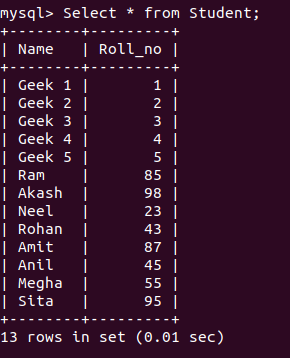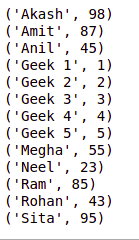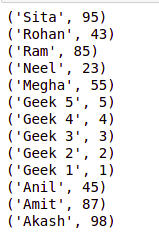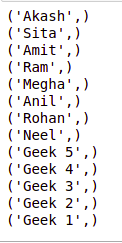Related Articles
Python MySQL – Order By Clause
• Last Updated : 09 Mar, 2020

A connector is employed when we have to use MySQL with other programming languages. The work of MySQL-connector is to provide access to MySQL Driver to the required language. Thus, it generates a connection between the programming language and the MySQL Server.

## OrderBy Clause

OrderBy is used to arrange the result set in either ascending or descending order. By default, it is always in ascending order unless “DESC” is mentioned, which arranges it in descending order.
“ASC” can also be used to explicitly arrange it in ascending order. But, it is generally not done this way since default already does that.

Syntax-

```SELECT column1, column2
FROM table_name
ORDER BY column_name ASC|DESC;
```

DATABASE IN USE:Example 1: Program to arrange the data in ascending order by name

 `# Python program to demonstrate``# order by clause`` ` ` ` `import` `mysql.connector`` ` `# Connecting to the Database``mydb ``=` `mysql.connector.connect(``  ``host ``=``'localhost'``,``  ``database ``=``'College'``,``  ``user ``=``'root'``,``  ``password ``=` `''``)`` ` `cs ``=` `mydb.cursor()`` ` `# Order by clause``statement ``=``"SELECT * FROM Student ORDER BY Name"``cs.execute(statement)`` ` `result_set ``=` `cs.fetchall()`` ` `for` `x ``in` `result_set:``    ``print``(x)``     ` `# Disconnecting from the database``mydb.close()`

Output:Example 2: Arranging the database in descending order

 `# Python program to demonstrate``# order by clause`` ` ` ` `import` `mysql.connector`` ` `# Connecting to the Database``mydb ``=` `mysql.connector.connect(``  ``host ``=``'localhost'``,``  ``database ``=``'College'``,``  ``user ``=``'root'``,``)`` ` `cs ``=` `mydb.cursor()`` ` `# Order by clause``statement ``=``"SELECT * FROM Student ORDER BY Name DESC"``cs.execute(statement)`` ` `result_set ``=` `cs.fetchall()`` ` `for` `x ``in` `result_set:``    ``print``(x)``   ` `# Disconnecting from the database  ``mydb.close()`

Output:Example 3: Program to get namefrom the table, arranged in descending order by Roll no.

 `# Python program to demonstrate``# order by clause`` ` ` ` `import` `mysql.connector`` ` `# Connecting to the Database``mydb ``=` `mysql.connector.connect(``  ``host ``=``'localhost'``,``  ``database ``=``'College'``,``  ``user ``=``'root'``,``)`` ` `cs ``=` `mydb.cursor()`` ` `# Order by clause``statement ``=``"SELECT Name FROM Student ORDER BY Roll_no DESC"``cs.execute(statement)`` ` `result_set ``=` `cs.fetchall()`` ` `for` `x ``in` `result_set:``    ``print``(x)``     ` `# Disconnecting from the database``mydb.close()`

Output:Attention geek! Strengthen your foundations with the Python Programming Foundation Course and learn the basics.

To begin with, your interview preparations Enhance your Data Structures concepts with the Python DS Course. And to begin with your Machine Learning Journey, join the Machine Learning – Basic Level Course

My Personal Notes arrow_drop_up